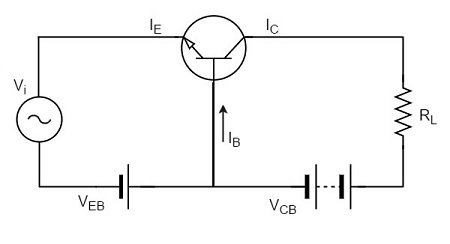# Transistor as an Amplifier

For a transistor to act as an amplifier, it should be properly biased. We will discuss the need for proper biasing in the next chapter. Here, let us focus how a transistor works as an amplifier.

## Transistor Amplifier

A transistor acts as an amplifier by raising the strength of a weak signal. The DC bias voltage applied to the emitter base junction, makes it remain in forward biased condition. This forward bias is maintained regardless of the polarity of the signal. The below figure shows how a transistor looks like when connected as an amplifier.The low resistance in input circuit, lets any small change in input signal to result in an appreciable change in the output. The emitter current caused by the input signal contributes the collector current, which when flows through the load resistor RL, results in a large voltage drop across it. Thus a small input voltage results in a large output voltage, which shows that the transistor works as an amplifier.

### Example

Let there be a change of 0.1v in the input voltage being applied, which further produces a change of 1mA in the emitter current. This emitter current will obviously produce a change in collector current, which would also be 1mA.

A load resistance of 5k&ohm; placed in the collector would produce a voltage of

5 k&ohm; Ã 1 mA = 5V

Hence it is observed that a change of 0.1v in the input gives a change of 5v in the output, which means the voltage level of the signal is amplified.

## Performance of Amplifier

As the common emitter mode of connection is mostly adopted, let us first understand a few important terms with reference to this mode of connection.

### Input Resistance

As the input circuit is forward biased, the input resistance will be low. The input resistance is the opposition offered by the base-emitter junction to the signal flow.

By definition, it is the ratio of small change in base-emitter voltage (ÎVBE) to the resulting change in base current (ÎIB) at constant collector-emitter voltage.

Input resistance, $R_i = \frac{\Delta V_{BE}}{\Delta I_B}$

Where Ri = input resistance, VBE = base-emitter voltage, and IB = base current.

### Output Resistance

The output resistance of a transistor amplifier is very high. The collector current changes very slightly with the change in collector-emitter voltage.

By definition, it is the ratio of change in collector-emitter voltage (ÎVCE) to the resulting change in collector current (ÎIC) at constant base current.

Output resistance = $R_o = \frac{\Delta V_{CE}}{\Delta I_C}$

Where Ro = Output resistance, VCE = Collector-emitter voltage, and IC = Collector-emitter voltage.

The load is connected at the collector of a transistor and for a single-stage amplifier, the output voltage is taken from the collector of the transistor and for a multi-stage amplifier, the same is collected from a cascaded stages of transistor circuit.

By definition, it is the total load as seen by the a.c. collector current. In case of single stage amplifiers, the effective collector load is a parallel combination of RC and Ro.

Effective Collector Load, $R_{AC} = R_C // R_o$

$$= \frac{R_C \times R_o}{R_C + R_o} = R_{AC}$$

Hence for a single stage amplifier, effective load is equal to collector load RC.

In a multi-stage amplifier (i.e. having more than one amplification stage), the input resistance Ri of the next stage also comes into picture.

Effective collector load becomes parallel combination of RC, Ro and Ri i.e,

Effective Collector Load, $R_{AC} = R_C // R_o // R_i$

$$R_C // R_i = \frac{R_C R_i}{R_C + R_i}$$

As input resistance Ri is quite small, therefore effective load is reduced.

### Current Gain

The gain in terms of current when the changes in input and output currents are observed, is called as Current gain. By definition, it is the ratio of change in collector current (ÎIC) to the change in base current (ÎIB).

Current gain, $\beta = \frac{\Delta I_C}{\Delta I_B}$

The value of Î² ranges from 20 to 500. The current gain indicates that input current becomes Î² times in the collector current.

### Voltage Gain

The gain in terms of voltage when the changes in input and output currents are observed, is called as Voltage gain. By definition, it is the ratio of change in output voltage (ÎVCE) to the change in input voltage (ÎVBE).

Voltage gain, $A_V = \frac{\Delta V_{CE}}{\Delta V_{BE}}$

$$= \frac{Change \: in\: output \: current \times effective\: load}{Change \: in\: input \: current \times input \: resistance}$$

$$= \frac{\Delta I_C \times R_{AC}}{\Delta I_B \times R_i} = \frac{\Delta I_C}{\Delta I_B} \times \frac{R_{AC}}{R_i} = \beta \times \frac{R_{AC}}{R_i}$$

For a single stage, RAC = RC.

However, for Multistage,

$$R_{AC} = \frac{R_C \times R_i}{R_C + R_i}$$

Where Ri is the input resistance of the next stage.

### Power Gain

The gain in terms of power when the changes in input and output currents are observed, is called as Power gain.

By definition, it is the ratio of output signal power to the input signal power.

Power gain, $A_P = \frac{(\Delta I_C)^2 \times R_{AC}}{(\Delta I_B)^2 \times R_i}$

$$= \left ( \frac{\Delta I_C}{\Delta I_B} \right ) \times \frac{\Delta I_C \times R_{AC}}{\Delta I_B \times R_i}$$

= Current gain Ã Voltage gain

Hence these are all the important terms which refer the performance of amplifiers.